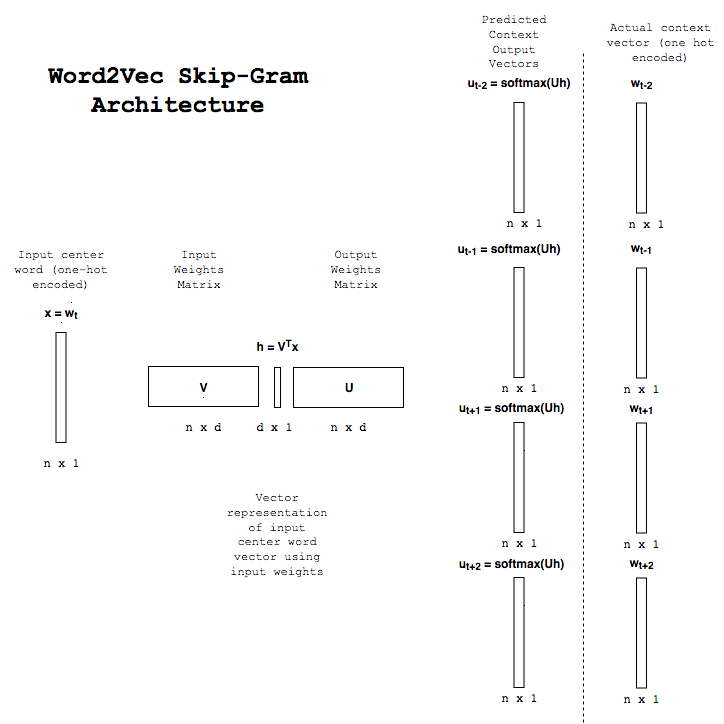# Word2Vec: Skip-Gram Feedforward Architecture

Reading through papers on the Word2vec skip-gram model, I found myself confused on a fairly uninteresting point in the mechanics of the output layer. What was never made explicit enough (at least to me) is that the output layer returns the exact same output distribution for each context.

To see why this must be true, notice that for a given one-hot encoded vector $x$, its hidden layer representation is $h=Wx$ where $W$ is the input weight matrix. To compute the output of the network, we compute $\mathrm{softmax}(U^Th)$ where $U$ is the output weight matrix. But this is completely independent of the context word we are trying to predict. Therefore, it must be constant for each context. Diagrams of the model, e.g. as presented here, tend to make this a bit confusing since they seem to suggest that multiple vectors are being generated for a given input.

The reason these diagrams are drawn this way is, of course, for the sake of error propagation. Although the output context vectors are the same for each input, the error in predicting the actual context word using the context vector will be quite different. So the output layer is better viewed as copying the same output to multiple different “panels”. In each panel, the error computation will be different but the predicted value is still the same.

For the sake of my own sanity, I redrew the feedforward architecture diagram.Hopefully, this post will help others spend less time on this detail of word2vec than I have.